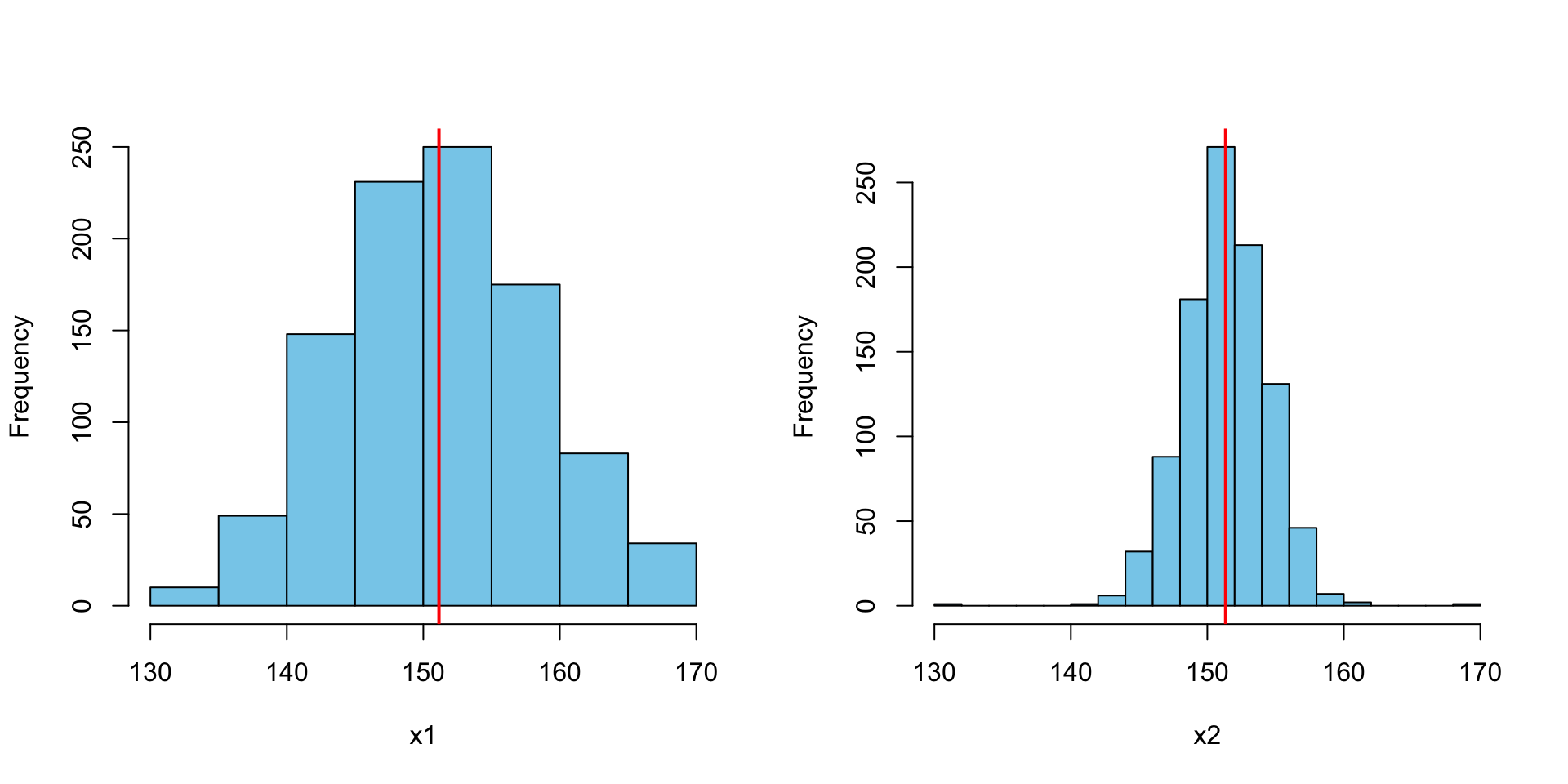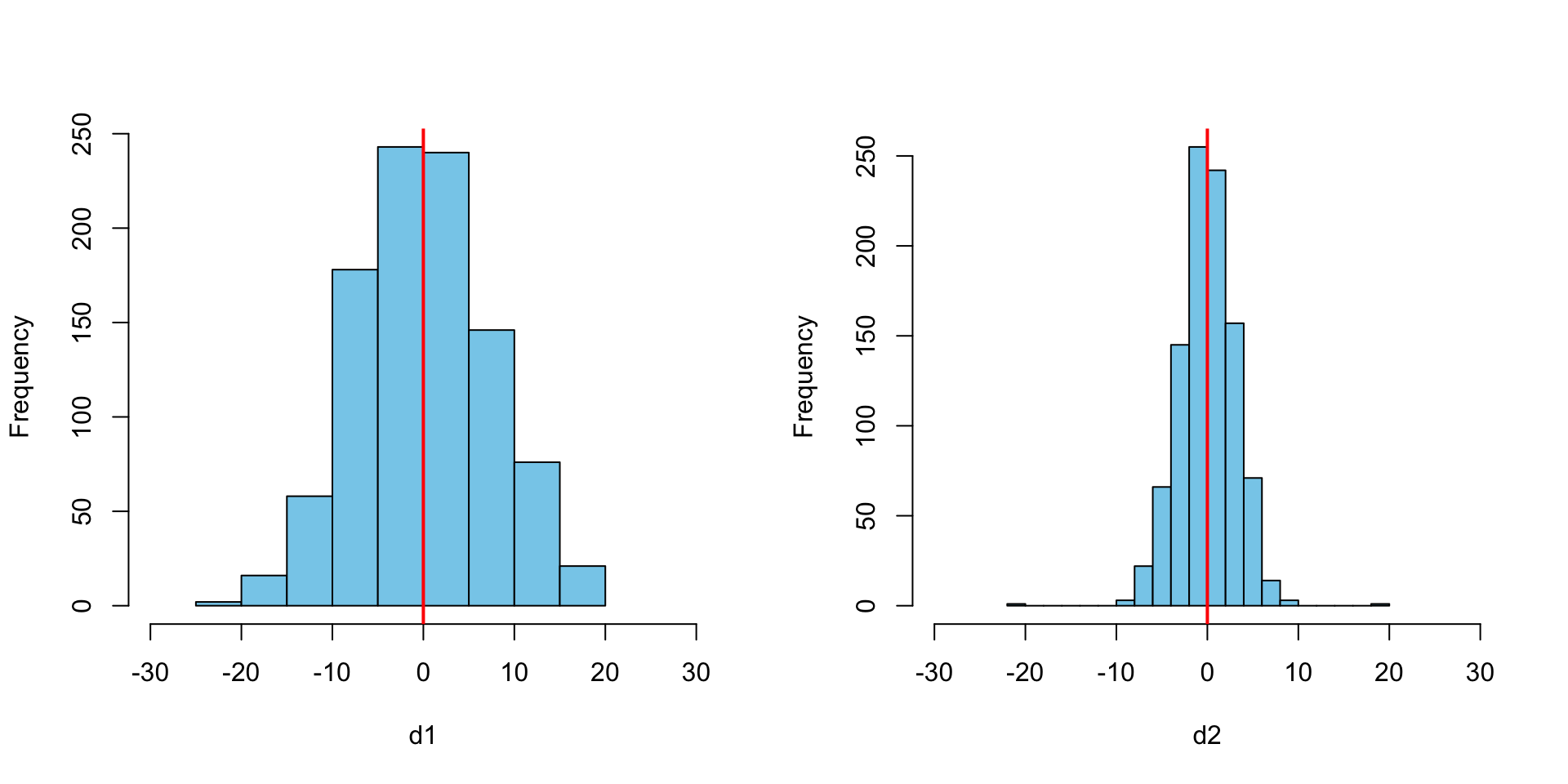# Measures of Variability

While central tendency is important, we often want to understand more than just were the center of a distribution is located, we often also find useful measures of the spread of the values around the center. Such statistics are referred to as measures of dispersion or variability. Common measures I will discuss in this tutorial include the range, interquartile range, variance, and standard deviation.

William Murrah www.statistical-thinking.com (QMER)aub.ie/qmer
2020-09-23

## Packages Used

I use the following packages in this tutorial.

library(psych)
library(ggplot2)


## Important Notation

Scientific notation, while often confusing and frustrating initially, is very useful in helping to convey complex ideas in a compact and precise manner. The table below contains scientific notations relevant to measures of variability.

Symbol meaning
$$IQR$$ Interquartile range
$$\sigma^2$$ Population variance
$$s^2$$ sample variance
$$\sigma$$ Population standard deviation
$$s$$ sample standard deviation

## Measures of Variability or Dispursion

• A measure of central tendency is not sufficient to describe a numerical variable.
• In addition to information about the typical value, we also want to know how scores vary (or are dispersed) around the typical score.

## Range

We need some way to quantify the variability of the scores in distributions that reflects all scores and is sensitive to outliers. We already have a single score for the average or “typical” score - the mean. Ideally, we want a single number that represents the typical variation from the mean. The range is the simplest way to describe variability or how scores are dispersed across possible values. The range is the difference between the highest and lowest values in the variable.

 Range = Highest - Lowest
• The range is useful for identifying outliers.
• But it is also very sensitive to outliers.
• If one value is drastically different for the others, the range can be misleading.
• For example, see the histogram of GRE scores above.
• This makes a major limitation of the range apparent: it is based on only two of the scores in the variable.

### Example

GRE scores for two classes with the same mean (151.3) and same range (40):

set.seed(1234)
x1 <- rnorm(1000, 151.3, 8)
x1 <- x1[x1 > 130 & x1 < 170]
x2 <- c(rnorm(length(x1)-2, 151.3, 3), 130, 170)
par(mfrow = c(1,2))
hist(x1, col = "skyblue", xlim = c(130, 170), main = NULL)
abline(v = mean(x1), col = "red", lwd = 2)
hist(x2, col = "skyblue", xlim = c(130, 170), main = NULL, breaks = 15)
abline(v = mean(x2), col = "red", lwd = 2)par(mfrow = c(1, 1))

range(x1)

 130 169
range(x2)

 130 170

## Quantiles

• Quantiles are a set of values in a variable that divide it into equal groups.
• The most common is quartiles, which divide a variable into four equal parts, so that there are the same number of scores in each quartile.
• The lower or first quartile separates the lower 25% of the scores from the upper 75%,
• the second or median quartile – which is the median value – separates the lower and upper 50%,
• and the third or upper quartile separates the lower 75% from the upper 25%.
• These three quartiles separate the variable into 4 equal parts.

For example, we can get the quartiles of using the quantiles() function in R. Here I do this for x1above.

quantile(x1)

  0%  25%  50%  75% 100%
130  146  151  156  169 
quantile(x2)

  0%  25%  50%  75% 100%
130  149  151  153  170 

## Interquartile Range

The interquartile range is simply the range of values from the $$25^{th}$$ and $$75^{th}$$ quartiles. A benefit of this measures is that is is not as sensitive to outliers as the range. For the above example, the interquartile range is 146 - 156. Notice that the $$50^{th}$$ quantile, which is the second quartile, is the median:

quantile(x1, probs = c(.25, .75))

25% 75%
146 156 
median(x1)

 151

## Variance

A major limitation of the range and interquartile range is that they are calculated with only two values in the data. What we really want is a measure of dispersion that takes into consideration all the values in the variable. The variance does this. The variance is a very important measure of variability. It is also closely related to the standard deviation, which we talk about next. In words the variance is the average squared deviation But let’s try to understand why we calculate the variance the way we do. To do that we need to understand deviations.

### Deviance

We can calculate the distance between the mean and each score. These distances are called deviations. Each score has a deviation. I have plotted the histogram of the deviations for each of the two classes GRE scores below.

par(mfrow = c(1,2))
d1 <- x1 - mean(x1)
d2 <- x2 - mean(x2)
hist(d1, col = "skyblue", xlim = c(-30, 30), main = NULL)
abline(v = mean(d1), col = "red", lwd = 2)
hist(d2, col = "skyblue", xlim = c(-30, 30), main = NULL, breaks = 15)
abline(v = mean(d2), col = "red", lwd = 2)par(mfrow = c(1, 1))


We might think to just take the mean of the deviations as a measure of the average or “typical” distance from the mean for each set of scores. But, the mean deviation for the first score is 0 and the mean deviation for the second set is 0. This is because, being a measure of central tendency, the mean is in the middle, and the positive distances of scores above the mean cancel out the negative distances below the mean.

We could take the mean of the absolute values of the deviations, but a more ingenious solution is to square the deviations.

This does two things:

1. Squaring takes care of the problem of the deviations summing to 0, as all the squared deviations will be positive. Remember, positive times a positive is a positive, but a negative times a negative is also a positive.
2. Because deviations are squared, numbers further from the mean have a greater influence than those closer to the mean. Therefore, deviations are sensitive to outliers. This can be good or bad, depending on the situation.

The sum of the squared deviations is often referred to simply as the “sum of squares”, and symbolized as $$SS$$. The sum of squares is not very meaningful by itself, so we often calculate the mean squared deviation, by dividing the $$SS$$ by $$N$$. This give us:

### Population Variance

$\sigma^2 = \frac{SS}{N} = \frac{\Sigma(x - \mu)^2}{N}$

### Sample Variance

$s^2 = \frac{\Sigma{(x - \bar{X})^2}}{n - 1}$

## Comparing formulae for mean and variance

$\mu = \frac{\Sigma x}{N}, \quad \sigma^2 = \frac{\Sigma(x - \mu)^2}{N}.$

Comparing the formulae for the mean and variance makes clear that the variance is the mean squared deviation.

## Standard Deviation

If the variance is the mean squared distance from the mean, then taking the square root of the variance gives us the mean, or average, distance from the mean.

### Population standard deviation

$\sigma = \sqrt{\sigma^2} = \sqrt{\frac{SS}{N}} = \sqrt{\frac{\Sigma{(x -\mu)^2}}{N}}.$

### Sample standard deviation

$s = \sqrt{s^2} = \sqrt{\frac{SS}{n-1}} = \sqrt{\frac{\Sigma{(x - M)^2}}{n-1}}.$

## Standard Deviation

par(mfrow = c(1,2))
hist(x1, col = "skyblue", xlim = c(130, 170), main = NULL)
abline(v = mean(x1), col = "red", lwd = 2)
hist(x2, col = "skyblue", xlim = c(130, 170), main = NULL, breaks = 15)
abline(v = mean(x2), col = "red", lwd = 2)par(mfrow = c(1, 1))


The variance of x1 is 54.71 and the variance of x2 is 9.51. The standard deviation of x1 is 7.4 and the standard deviation of x2 is 3.08.

## Standard Deviation

d <- data.frame(x1, x2)
describe(d, fast = TRUE)

   vars   n mean  sd min max range   se
x1    1 980  151 7.4 130 169    39 0.24
x2    2 980  151 3.1 130 170    40 0.10